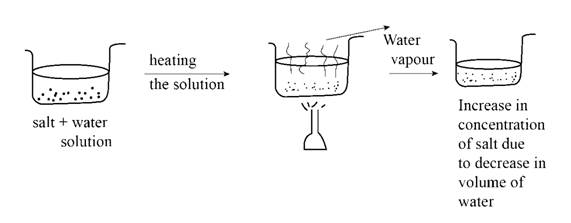# The change in salt concentration as the solution of table salt in water boils is to be stated. Concept Introduction: Solution may be defined as the mixture of a minor component which is known as solute and a major component known as solvent. The solute in the solution is uniformly distributed in the solvent. On heating a solution, the liquid generally boils and goes into the air and the salt remains in the remaining solvent as it is nonvolatile.### Introductory Chemistry: A Foundati...

9th Edition
Steven S. Zumdahl + 1 other
Publisher: Cengage Learning
ISBN: 9781337399425### Introductory Chemistry: A Foundati...

9th Edition
Steven S. Zumdahl + 1 other
Publisher: Cengage Learning
ISBN: 9781337399425

#### Solutions

Chapter 15, Problem 1ALQ
Interpretation Introduction

## Interpretation:The change in salt concentration as the solution of table salt in water boils is to be stated.Concept Introduction:Solution may be defined as the mixture of a minor component which is known as solute and a major component known as solvent. The solute in the solution is uniformly distributed in the solvent. On heating a solution, the liquid generally boils and goes into the air and the salt remains in the remaining solvent as it is nonvolatile.

Expert Solution

The concentration of the salt increases as the solution boils.

### Explanation of Solution

Table salt mixed with water forms a homogeneous solution. When the solution is heated to the boiling point of water, water in the solution boils and goes in the air. Salt in the solution is nonvalatile and does not boils and remain as it is in the solution. Due to this reason, the volume of water decreases and the salt concentration in the solution increases as shown in Figure 1.Figure 1.

Conclusion

The concentration of the salt increases as the solution boils.

### Want to see more full solutions like this?

Subscribe now to access step-by-step solutions to millions of textbook problems written by subject matter experts!

### Want to see more full solutions like this?

Subscribe now to access step-by-step solutions to millions of textbook problems written by subject matter experts!